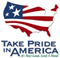# CALCULATION OF INDIVIDUAL ISOTOPE EQUILIBRIUM CONSTANTS FOR IMPLEMENTATION IN GEOCHEMICAL MODELS

## Abstract

Theory is derived from the work of Urey to calculate equilibrium constants commonly used in geochemical equilibrium and reaction-transport models for reactions of individual isotopic species. Urey showed that equilibrium constants of isotope exchange reactions for molecules that contain two or more atoms of the same element in equivalent positions are related to isotope fractionation factors by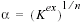, where is n the number of atoms exchanged. This relation is extended to include species containing multiple isotopes, for example and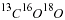, and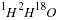to include the effects of nonideality. The equilibrium constants of the isotope exchange reactions provide a basis for calculating the individual isotope equilibrium constants for the geochemical modeling reactions. The temperature dependence of the individual isotope equilibrium constants can be calculated from the temperature dependence of the fractionation factors. Equilibrium constants are calculated for all species that can be formed from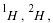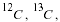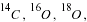and selected species containing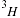, in the molecules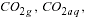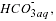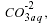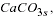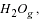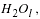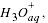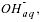and the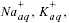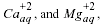ion pairs with where the subscripts g, aq, l, and s refer to gas, aqueous, liquid, and solid, respectively. These equilibrium constants are used in the geochemical model PHREEQC to produce an equilibrium and reaction-transport model that includes these isotopic species. Methods are presented for calculation of the individual isotope equilibrium constants for the asymmetric bicarbonate ion. An example calculates the equilibrium of multiple isotopes among multiple species and phases.

## CONTENTS

Abstract

Introduction

Isotope ratios in gaseous and aqueous CO2

Species and symmetry numbers

Equilbrium constants for intraspecies reactions among the CO2 species

Isotope ratios

Calculation of individual isotope equilibrium constants for the reaction CO2gas - CO2aqueous

CO18O

C18O2

13CO2

13CO18O

13C18O2

14CO2, 14CO18O, and 14C18O2

Symmetry assumptions in the isotope ratios

Individual isotope equilibrium constants for bicarbonate

Individual isotope equilibrium constants for ion pairs

Application to other isotopes

Nonideality

Temperature dependence

Fundamental individual isotope equilibrium constants

Comparison of methods

Example calculation using PHREEQC

Summary

References cited

The text and graphics are presented here in pdf format (print quality):

The full report is 912KB

 AccessibilityFOIAPrivacyPolicies and Notices U.S. Department of the Interior, U.S. Geological Survey Persistent URL: http://pubsdata.usgs.gov/pubs/wri/wrir02-4172/ Page Contact Information: USGS Publishing Network Last modified: Wednesday, December 07 2016, 01:12:34 PM